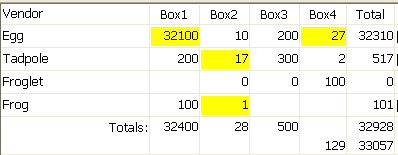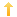## PowerBuilder – Change Background of Modified Columns in Datawindow

Posted on Thursday, June 17th, 2010 at 8:20 pm in

Okay so you have a datawindow with a bunch of rows which can be edited (a grid datawindow is a good choice).  So when the user pulls up the data set and proceeds to change values in a variety of columns and a variety of rows you want to indicate to him that the specific row/column has been changed by altering the background color.

I have a solution of sorts which, once you get it going, will work.  It involves:
1) adding a column on the datawindow object
2) populating that column in the itemchanged event
3) putting an expression on the background color property of each visible column in the datawindow object you want the color to change when edited

1) I added a string column called ‘change’ (not a computed col) to my datawindow object.

2) in the Itemchanged event on the Datawindow

```   // build a list of the column numbers which have been edited.
// You can enhance this to account for errors, etc.
string ls_desc, ls_value, ls_d, ls_prev
integer li_rc
ls_desc =  dwo.name + '.ID'
ls_d = describe(ls_desc)
ls_prev = getitemstring(row,'change')
IF IsNull(ls_prev) THEN ls_prev = ''
ls_value = ls_prev + '[' + ls_d + ']' // append new value
li_rc =    this.setitem( row, 'change', ls_value)
```

3) in the background color of a column called ‘box3’ put the following expression

`IF (pos(change, '[' + describe('box3.ID') + ']') > 0, RGB(255,255,0),RGB(255,255,255))`

As an alternative, you can make step three dynamic in an open/postopen type event:

```dw_1.retrieve( )
dw_1.setredraw( False)
// get list of columns in the order they are displayed which may not be the same as their column number order
ll_max = Integer(dw_1.Object.DataWindow.Column.Count)  // determine number of columns
FOR ll_i = 1 TO ll_max STEP 1
// only need the visible columns
IF dw_1.describe("#" + String(ll_i) + ".Visible") = '1' THEN
// first set the mode to opaque
ls_modstring = dw_1.describe( "#" + string(ll_i) +  ".Name") +  ".Background.Mode='0'"
ls_msg = dw_1.Modify(ls_modstring)
// set the color of the cells
ls_modstring = dw_1.describe( "#" + string(ll_i) +  ".Name") +  ".Background.Color =  ~"536870912~t IF (pos(change,~'[~' + describe(~'" + &
dw_1.describe( "#" + string(ll_i) +  ".Name") + ".ID~') + ~']~') > 0, RGB(255,255,0),RGB(255,0,255))~""

ls_msg = dw_1.Modify(ls_modstring)
END IF

NEXT

dw_1.setRedraw(true)
```

Sample view:You might also be interested in
Tags: